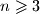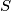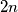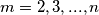### IMO Shortlist 2018 problem C1

Kvaliteta:
Avg: 0,0
Težina:
Avg: 6,0

Let$n\geqslant 3$ be an integer. Prove that there exists a set$S$ of$2n$ positive integers satisfying the following property: For every$m=2,3,...,n$ the set$S$ can be partitioned into two subsets with equal sums of elements, with one of subsets of cardinality$m$.

Izvor: https://www.imo-official.org/problems/IMO2018SL.pdf